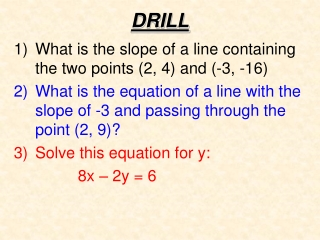DownloadDownload PresentationDRILL

# DRILL

Download Presentation## DRILL

- - - - - - - - - - - - - - - - - - - - - - - - - - - E N D - - - - - - - - - - - - - - - - - - - - - - - - - - -
##### Presentation Transcript

1. DRILL • What is the slope of a line containing the two points (2, 4) and (-3, -16) • What is the equation of a line with the slope of -3 and passing through the point (2, 9)? • Solve this equation for y: 8x – 2y = 6

2. 8 6 4 2 6 8 -2 -8 -6 -4 2 4 -2 -4 -6 -8 Parallel Lines Parallel lines are lines in the same plane that never intersect. Parallel lines have the same slope.

3. 8 6 4 2 6 8 -2 -8 -6 -4 2 4 -2 -4 -6 -8 Perpendicular Lines Perpendicular lines are lines that intersect to form a 900 angle. The product of the slopes of perpendicular lines is -1.

4. Example 1 Determine whether these lines are parallel or perpendicular. and -15x + 3y = 9 y – 2 = 5x + 4 y = 5x + 6 3y = 9 + 15x y = 3 + 5x y = 5x + 3 The lines have the same slope. So they are parallel.

5. Example 2 Write an equation in slope-intercept form for the line containing (-3,-5) and parallel to the line y = 2x + 1. m = 2 First, we need the slope of the line y = 2x + 1. Second, we need to find out the slope of the line that is parallel to y = 2x + 1. Lastly, we use the point-slope formula to find our equation. y + 5 = 2x + 6 y = 2x + 1

6. Example 3 Write an equation for the line containing (-3,-5) and perpendicular to the line y = 2x + 1. First, we need the slope of the line y = 2x + 1. m = 2 Second, we need to find out the slope of the line that is perpendicular to y = 2x + 1. Lastly, we use the point-slope formula to find our equation.

7. Concept – Parallel Lines Two nonvertical lines are parallel if they have the same slope. In other words, if the slope of one line is and the slope of the other line is the two lines are parallel if If two lines are both vertical lines, they are parallel.

8. Example - Parallel Lines Are the two lines parallel? & The slope of the first line is - 4. The slope of the second line is also - 4. The lines are parallel.

9. Concept – Perpendicular Lines Two nonvertical lines are perpendicular if their slopes are negative reciprocals. In other words, if the slope of one line is and the slope of the other line is the two lines are perpendicular if A vertical line is perpendicular to a horizontal line.

10. Example - Perpendicular Lines Are the two lines perpendicular ? & The slope of the first line is The slope of the second line is The lines are perpendicular.

11. Concept – Point-Slope Form of a Line The point-slope form of the equation of a line with slope m that passes through the point is This form of a line is used to find the equation of a line if its slope and the coordinates of a point on the line are known.